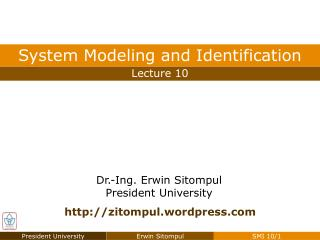# Homework 9 - PowerPoint PPT PresentationDownload PresentationHomework 9

Homework 9Download Presentation## Homework 9

- - - - - - - - - - - - - - - - - - - - - - - - - - - E N D - - - - - - - - - - - - - - - - - - - - - - - - - - -
##### Presentation Transcript

1. Chapter 6 Identification from Step Response Homework 9 • Time Percent Value MethodDetermine the approximation of the model in the last example, if after examining the t/t table, the model order is chosen to be 4 instead of 5.

2. Solution of Homework 9 Chapter 6 Identification from Step Response 5 values of ti/τ are to be located for n = 4 Result: t/τ Table

3. Chapter 6 Identification from Step Response Solution of Homework 9 : 5th order approximation : 4th order approximation

4. Chapter 6 Least Squares Methods Least Squares Methods • The Least Squares Methods are based on the minimization of squares of errors. • The errors are defined as the difference between the measured value and the estimated value of the process output, or between y(k) and y(k). • There are two version of the methods: batch version and recursive version. ^

5. Chapter 6 Least Squares Methods Least Squares Methods • Consider the discrete-time transfer function in the form of: • The aim of Least Squares (LS) Methods is to identify the parameters a1, ..., an, b1, ..., bm from the knowledge of process inputs u(k) and process output y(k). • As described by the transfer function above, the relation of process inputs and process outputs is:

6. Chapter 6 Least Squares Methods Least Squares Methods • This relation can be written in matrix notation as: where: • Vector of Parameters • Vector of Measured Data • Hence, the identification problem in this case is how to find θ based on the actual process output y(k) and the measured data from the past m(k).

7. Chapter 6 Least Squares Methods Least Squares Methods • Assuming that the measurement was done for k times, with k ≥ n + m, then k equations can be constructed as follows: or:

8. Chapter 6 Least Squares Methods Least Squares Methods • If M is nonsingular, then the direct solution can be calculated as: • Least Error (LE) Method, Batch Version • In this method, error is minimized as a linear function of the parameter vector. • The disadvantage of this solution is, that error can be abruptly larger for t > k.

9. Chapter 6 Least Squares Methods Least Squares Methods • A better way to calculate the parameter estimate θ is to find the parameter set that will minimize the sum of squares of errors between the measured outputs y(k) and the model outputs y(k) = mT(k)θ ^ • The extreme of J with respect to θ is found when:

10. Chapter 6 Least Squares Methods Least Squares Methods • The derivation of J(θ) with respect to θ can be calculated as: • Least Squares (LS) Method, Batch Version if A symmetric

11. Chapter 6 Least Squares Methods Least Squares Methods • Performing the “Second Derivative Test”, Always positive definite is a solution that will minimize the squares of errors Second Derivative Test • If f’(x) = 0 and f”(x) > 0 then f has a local minimum at x • If f’(x) = 0 and f”(x) < 0 then f has a local maximum at x • If f’(x) = 0 and f”(x) = 0 then no conclusion can be drawn

12. Chapter 6 Least Squares Methods Least Squares Methods • In order to guarantee that MTM is invertible, the number of row of M must be at least equal to the number of its column, which is again the number of parameters to be identified. • More row of M increase the accuracy of the calculation. In other words, the number of data row does not have to be the same as the sum of the order of numerator and denominator of the model to be identified. • If possible, rows with any value assumed to be zero (because no measurement data exist) should not be used.

13. Chapter 6 Least Squares Methods Example: Least Squares Methods • The parameters of a model with the structure of: are to be identified out of the following measurement data: • Perform the batch version of the Least Squares Methods to find out a1, a2, and b2. • Hint: n + m = 2 + 1  At least 3 measurements must be available / utilized. Hint: If possible, avoid to many zeros due to unavailable data for u(k) = 0 and y(k) = 0, k < 0.

14. Chapter 6 Least Squares Methods Example: Least Squares Methods • Using the least allowable data, from k = 2 to k = 4, the matrices Y and M can be constructed as:

15. Chapter 6 Least Squares Methods Example: Least Squares Methods

16. Chapter 6 Least Squares Methods Homework 10 • Redo the example, utilizing as many data as possible. • Does your result differ from the result given in the slide? • What could be the reason for that? Which result is more accurate?

17. Chapter 6 Least Squares Methods Homework 10 • Redo the example, utilizing least allowable data, if the structure of the model is chosen to be NEW • After you found the three parameters a1, a2, and b1, for G2(z), use Matlab/Simulink to calculate the response of both G1(z) and G2(z) if they are given the sequence of input as given before. • Compare y(k) with y1(k) and y2(k)? Give analysis and conclusions.